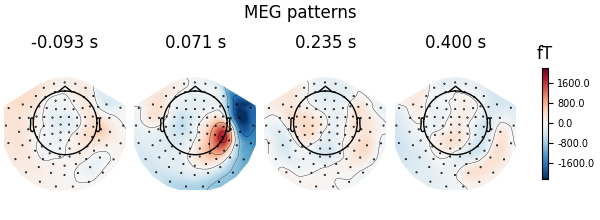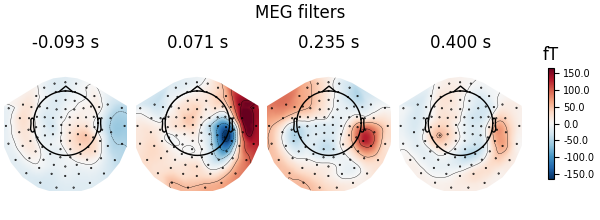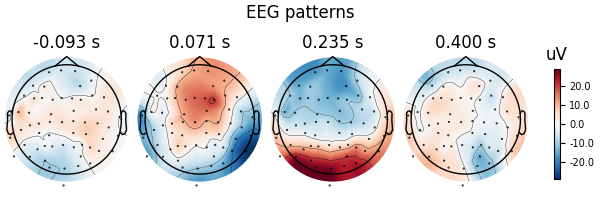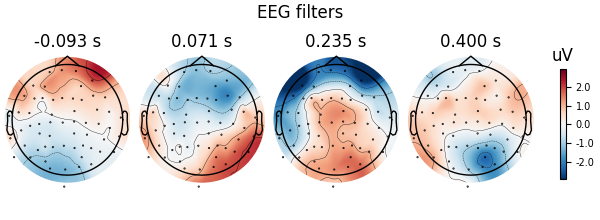# Linear classifier on sensor data with plot patterns and filters#

Here decoding, a.k.a MVPA or supervised machine learning, is applied to M/EEG data in sensor space. Fit a linear classifier with the LinearModel object providing topographical patterns which are more neurophysiologically interpretable  than the classifier filters (weight vectors). The patterns explain how the MEG and EEG data were generated from the discriminant neural sources which are extracted by the filters. Note patterns/filters in MEG data are more similar than EEG data because the noise is less spatially correlated in MEG than EEG.

```# Authors: Alexandre Gramfort <alexandre.gramfort@inria.fr>
#          Romain Trachel <trachelr@gmail.com>
#          Jean-Remi King <jeanremi.king@gmail.com>
#
```
```import mne
from mne import io, EvokedArray
from mne.datasets import sample
from mne.decoding import Vectorizer, get_coef

from sklearn.preprocessing import StandardScaler
from sklearn.linear_model import LogisticRegression
from sklearn.pipeline import make_pipeline

# import a linear classifier from mne.decoding
from mne.decoding import LinearModel

print(__doc__)

data_path = sample.data_path()
sample_path = data_path / 'MEG' / 'sample'
```

Set parameters

```raw_fname = sample_path / 'sample_audvis_filt-0-40_raw.fif'
event_fname = sample_path / 'sample_audvis_filt-0-40_raw-eve.fif'
tmin, tmax = -0.1, 0.4
event_id = dict(aud_l=1, vis_l=3)

# Setup for reading the raw data
raw.filter(.5, 25, fir_design='firwin')

epochs = mne.Epochs(raw, events, event_id, tmin, tmax, proj=True,
del raw

labels = epochs.events[:, -1]

# get MEG and EEG data
meg_epochs = epochs.copy().pick_types(meg=True, eeg=False)
meg_data = meg_epochs.get_data().reshape(len(labels), -1)
```
```Opening raw data file /home/circleci/mne_data/MNE-sample-data/MEG/sample/sample_audvis_filt-0-40_raw.fif...
Read a total of 4 projection items:
PCA-v1 (1 x 102)  idle
PCA-v2 (1 x 102)  idle
PCA-v3 (1 x 102)  idle
Average EEG reference (1 x 60)  idle
Range : 6450 ... 48149 =     42.956 ...   320.665 secs
Reading 0 ... 41699  =      0.000 ...   277.709 secs...
Filtering raw data in 1 contiguous segment
Setting up band-pass filter from 0.5 - 25 Hz

FIR filter parameters
---------------------
Designing a one-pass, zero-phase, non-causal bandpass filter:
- Windowed time-domain design (firwin) method
- Hamming window with 0.0194 passband ripple and 53 dB stopband attenuation
- Lower passband edge: 0.50
- Lower transition bandwidth: 0.50 Hz (-6 dB cutoff frequency: 0.25 Hz)
- Upper passband edge: 25.00 Hz
- Upper transition bandwidth: 6.25 Hz (-6 dB cutoff frequency: 28.12 Hz)
- Filter length: 993 samples (6.613 sec)

[Parallel(n_jobs=1)]: Using backend SequentialBackend with 1 concurrent workers.
[Parallel(n_jobs=1)]: Done   1 out of   1 | elapsed:    0.0s remaining:    0.0s
[Parallel(n_jobs=1)]: Done   2 out of   2 | elapsed:    0.0s remaining:    0.0s
[Parallel(n_jobs=1)]: Done   3 out of   3 | elapsed:    0.0s remaining:    0.0s
[Parallel(n_jobs=1)]: Done   4 out of   4 | elapsed:    0.0s remaining:    0.0s
[Parallel(n_jobs=1)]: Done 366 out of 366 | elapsed:    0.6s finished
145 matching events found
No baseline correction applied
Created an SSP operator (subspace dimension = 4)
4 projection items activated
Using data from preloaded Raw for 145 events and 76 original time points (prior to decimation) ...
Removing projector <Projection | Average EEG reference, active : True, n_channels : 60>
```

## Decoding in sensor space using a LogisticRegression classifier#

```clf = LogisticRegression(solver='liblinear')  # liblinear is faster than lbfgs
scaler = StandardScaler()

# create a linear model with LogisticRegression
model = LinearModel(clf)

# fit the classifier on MEG data
X = scaler.fit_transform(meg_data)
model.fit(X, labels)

# Extract and plot spatial filters and spatial patterns
for name, coef in (('patterns', model.patterns_), ('filters', model.filters_)):
# We fitted the linear model onto Z-scored data. To make the filters
# interpretable, we must reverse this normalization step
coef = scaler.inverse_transform([coef])

# The data was vectorized to fit a single model across all time points and
# all channels. We thus reshape it:
coef = coef.reshape(len(meg_epochs.ch_names), -1)

# Plot
evoked = EvokedArray(coef, meg_epochs.info, tmin=epochs.tmin)
fig = evoked.plot_topomap()
fig.suptitle(f'MEG {name}')
```
••Let’s do the same on EEG data using a scikit-learn pipeline

```X = epochs.pick_types(meg=False, eeg=True)
y = epochs.events[:, 2]

# Define a unique pipeline to sequentially:
clf = make_pipeline(
Vectorizer(),                       # 1) vectorize across time and channels
StandardScaler(),                   # 2) normalize features across trials
LinearModel(                        # 3) fits a logistic regression
LogisticRegression(solver='liblinear')
)
)
clf.fit(X, y)

# Extract and plot patterns and filters
for name in ('patterns_', 'filters_'):
# The `inverse_transform` parameter will call this method on any estimator
# contained in the pipeline, in reverse order.
coef = get_coef(clf, name, inverse_transform=True)
evoked = EvokedArray(coef, epochs.info, tmin=epochs.tmin)
fig = evoked.plot_topomap()
fig.suptitle(f'EEG {name[:-1]}')
```
••```Removing projector <Projection | PCA-v1, active : True, n_channels : 102>
Removing projector <Projection | PCA-v2, active : True, n_channels : 102>
Removing projector <Projection | PCA-v3, active : True, n_channels : 102>
```